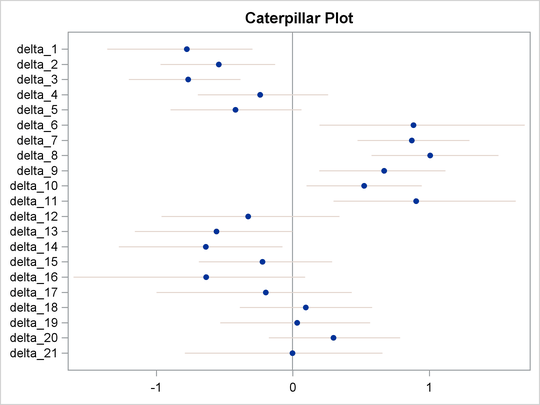The MCMC Procedure

Caterpillar Plot

The caterpillar plot is a side-by-side bar plot of 95% intervals for multiple parameters. Typically, it is used to visualize and compare random-effects parameters, which can come in large numbers in certain models. You can use the %CATER autocall macro to create a caterpillar plot. The %CATER macro requires you specify an input data set and a list of variables that you want to plot.

A random-effects model that has 21 random-effects parameters is used here for illustrational purpose. For an explanation of the random-effects model and the data involved, see Logistic Regression Random-Effects Model. The following statements generate a SAS data set and fit the model:

```title 'Create a Caterpillar Plot';

data seeds;
input r n seed extract @@;
ind = _N_;
datalines;
10  39  0  0    23  62  0  0    23  81  0  0    26  51  0  0
17  39  0  0     5   6  0  1    53  74  0  1    55  72  0  1
32  51  0  1    46  79  0  1    10  13  0  1     8  16  1  0
10  30  1  0     8  28  1  0    23  45  1  0     0   4  1  0
3  12  1  1    22  41  1  1    15  30  1  1    32  51  1  1
3   7  1  1
;
```
```ods select none;
proc mcmc data=seeds outpost=postout seed=332786 nmc=20000;
parms beta0 0 beta1 0 beta2 0 beta3 0 s2 1;
prior s2 ~ igamma(0.01, s=0.01);
prior beta: ~ general(0);
w = beta0 + beta1*seed + beta2*extract + beta3*seed*extract;
random delta ~ normal(w, var=s2) subject=ind;
pi = logistic(delta);
model r ~ binomial(n = n, p = pi);
run;
ods select all;
```

The output data set `Postout` contains posterior draws for all 21 random-effects parameters, `delta_1``delta_21`. You can use the following statements to generate a caterpillar plot for the 21 parameters:

```%CATER(data=postout, var=delta:);
```

Figure 73.18 is a caterpillar plot of the random-effects parameters `delta_1``delta_21`.

Figure 73.18: Caterpillar Plot of the Random-Effects ParametersIf you want to change the display of the caterpillar plot, such as using a different line pattern, color, or size of the markers, you need to first modify the `Stat.MCMC.Graphics.Caterpillar` template and then call the %CATER macro again.

You can use the following statements to view the source of the `Stat.MCMC.Graphics.Caterpillar` template:

```proc template;
path sashelp.tmplmst;
source Stat.MCMC.Graphics.Caterpillar;
run;
```

Figure 73.19 lists the source statements of the template that is used to generate the template for the caterpillar plot.

Figure 73.19: Source Statements for Stat.MCMC.Graphics.Caterpillar Template

 ```define statgraph Stat.MCMC.Graphics.Caterpillar; dynamic _OverallMean _VarName _VarMean _XLower _XUpper _byline_ _bytitle_ _byfootnote_; begingraph; entrytitle "Caterpillar Plot"; layout overlay / yaxisopts=(offsetmin=0.05 offsetmax=0.05 display=(line ticks tickvalues)) xaxisopts=(display=(line ticks tickvalues)); referenceline x=_OVERALLMEAN / lineattrs=(color= GraphReference:ContrastColor); HighLowPlot y=_VARNAME high=_XUPPER low=_XLOWER / lineattrs= GRAPHCONFIDENCE; scatterplot y=_VARNAME x=_VARMEAN / markerattrs=(size=5 symbol= circlefilled); endlayout; if (_BYTITLE_) entrytitle _BYLINE_ / textattrs=GRAPHVALUETEXT; else if (_BYFOOTNOTE_) entryfootnote halign=left _BYLINE_; endif; endif; endgraph; end; ```

You can use the TEMPLATE procedure (see Chapter 21: Statistical Graphics Using ODS) to modify the graph template. Subsequent calls to the %CATER macro will use the modified template to make the graph.